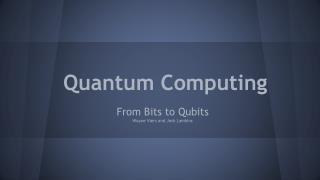DownloadDownload PresentationQuantum Computing

# Quantum Computing

Télécharger la présentation## Quantum Computing

- - - - - - - - - - - - - - - - - - - - - - - - - - - E N D - - - - - - - - - - - - - - - - - - - - - - - - - - -
##### Presentation Transcript

1. Quantum Computing From Bits to Qubits Wayne Viers and Josh Lamkins

2. Quantum Computing Algorithms • Shor’s Algorithm - integer factorization • Grovers Algorithm - searching an unsorted database

3. How Hard is Factoring? • RSA-129 - 129 Decimal Digits factored in 1994 using 1600 machines. • RSA-768 - 768 bits (232 decimal digits) factored in 2009 using ~2000 years of computational power.

4. Best Known Algorithms • b = log2n • Brute Force Method for i in range of (2, sqrt(n)) if is_factor(i,n) return i RSA modulus Running Time # bits Linear in the sqrt(n) O(2b/2) Assume Constant

5. Best Known Algorithms * • Best Known • General Number Field Sieve • Exponential Running Time • O(b1/3 log b2/3) • Best Known for Quantum Computer • Shor’s Algorithm * Classical Computer

6. Shor’s Algorithm • Named after mathematician Peter Shor. • Quantum Algorithm for integer factorization formulated in 1994. • Simply put, it solves the following problem: • “Given an integer N, find its prime factors.”

7. Shor’s Algorithm Efficiency • On a quantum computer, to factor an integer N, takes O((log N)3). • This demonstrates that integer factorization problems can be efficiently solved on a quantum computer.

8. Complexity Class • BQP - (Bounded-error Quantum Polynomial Time) is the class of decision problems solvable by a quantum computer in polynomial time, with an error probability of at most 1/3 for all instances.

9. Shor’s Algorithm - 2 Parts • A reduction, which can be done on a classicalcomputer, of the factoring problem to the problem of order-finding. • A quantum algorithm to solve the order-finding problem.

10. Shor’s Algorithm - Another Version • The classical part of the algorithm turns the factoring problem into the problem of finding the period of a function - this part may be implemented in a classic computer. • The second part finds the period using a Quantum Fourier Transform and is responsible for the quantum speedup.

11. Shor’s Algorithm - Classical Part • Pick a random number a<N • Compute gcd(a, N) (this may be done using the Euclidian Algorithm). • If gcd(a, N) 1, then there is a nontrivial factor of N and we are done. • Otherwise, use the period-finding subroutine to find r, the period of the following function: • f(x) = ax mod n • If r is odd, go back to step 1. • If ar/2= -1(mod N), go back to step 1. • gcd(ar/2+/- 1,N) is a nontrivial factor of N, we are done. Quantum Part of the Algorithm

12. Shor’s Algorithm - Quantum Part • The Fourier Transform relates a functions time domain with its frequency domain. The component frequencies spread across the frequency spectrum, are represented as peaks in the frequency domain.

13. Shor’s Algorithm - Quantum Part • Fourier analysis converts time (or space) to frequency and vice versa; a FFT rapidly computes such transformations by factorizing the DFT matrix into a product of sparse (mostly zero) factors. • The Quantum Fourier transform is the classical discrete Fourier transform applied to the vector of amplitudes of a quantum state.

14. Grover’s • Can be used to perform a search on an unsorted database • O(N1/2) run time with only O(logN) storage space • Has exponential speedup over the fastest possible classical algorithm

15. Grover’s • The algorithm more specifically inverts functions → Finds y given F(x) and vice versa • In the case of searching databases a function can be determined whose solution is an element in a database.

16. Other Grover’s Applications • Can be used to estimate the mean or median for a large set of numbers • Can be used to solve the collision problem (finding two inputs for a function that map to the same value)

17. Grover’s • A probabilistic algorithm • Probability of failure is decreased by running the algorithm many times

18. Grover’s Algorithm Steps • Setup • Unsorted database of N entries • logN qubits • Have a function that maps each database entry to 0 or 1, with only one index mapping to 1 • The qubits are initialized to all the possible function inputs

19. Grover’s Algorithm Steps • Takes a function and a set of its possible inputs • Searches through those inputs • Finds the single one that causes the algorithm to return true.

20. Phase Inversion

21. Mean Inversion

22. Grover’s Steps

23. Whole Algorithm Example